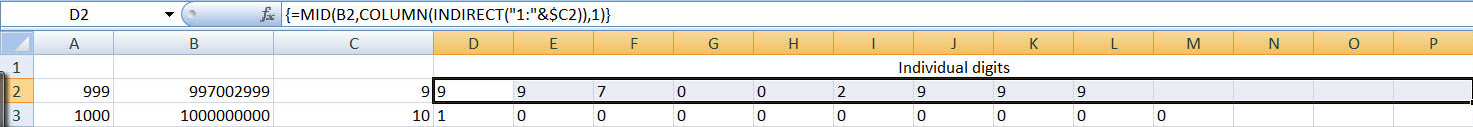﻿ Project Euler Problem 62
You are on the Home/Other Tutorials/Project Euler/Problem 62 pageShare Your

# Project Euler - Problem 62

## Problem description

The cube, 41063625 (3453), can be permuted to produce two other cubes: 56623104 (3843) and 66430125 (4053). In fact, 41063625 is the smallest cube which has exactly three permutations of its digits which are also cube.

Find the smallest cube for which exactly five permutations of its digits are cube.

## Solution

This is another problem that one can solve in Excel without any VBA support.

Before we can address the problem itself, we must address a basic question.  How does one figure out if one number is a permutation of the digits of another number?  I solved this with the following approach.  Map each number into a 10 character string (we could have used an array but it is a lot easier dealing with strings in Excel).  The position in the string represents a specific digit.  The character in that position represents the frequency with which that digit occurs in the number.  Hence, the first character (position 1) corresponds to the digit 0.  So, if the digit 0 occurs 4 times in the number the first character in the string will be 4.  To deal with frequencies greater than 9, I decided that the frequency would be stored using the hexadecimal system.  Now, to check if one number is a permutation of the digits of another number, all we have to do is check the corresponding digit maps.  If they are the same the numbers are permutations.  If not, they are not.

With this out of the way, we can address the specifics of the problem.  Since the description of the problem includes 3 permutations and 3 digit numbers, my guess was that five permutations would require at least a 4 digit number.  So, I picked the range 999 to 10,000.

An advantage of picking an upper limit of 10,000 is that 100003 is a 13 digit number.  Since Excel supports 15 digits of precision the range of interest is within the program's capabilities.

Next, we need to figure out how to map the cube of a number into its digit map and then check how often each digit map occurs in the range of numbers from 999 to 10,000.  The base number will be in column A starting with row 2.  In column B calculate the cube of the number.  In column C calculate the number of digits in the cube.  In the 13 columns D:P extract the individual digits of the cube.  In Q:Z calculate the frequency of each of the digits 0 through 9.  In AA concatenate the frequencies to get a single 10 character string.  This is the digit map for the cubed number.  In column AB calculate the frequency with which the digit map occurs.  Finally, in AC2 calculate the cubed value corresponding to a frequency of 5 in column AB.

Start by entering in A2 the value 999.

B2 contains the formula =A2^3.  C2 contains the formula =INT(LOG10(B2))+1 though it could just as easily contain the formula =LEN(B2).

D2:P2 contain the *array* formula =MID(B2,COLUMN(INDIRECT("1:"&\$C2)),1).  To enter an array formula complete the formula with the CTRL+SHIFT+ENTER key combination rather than just the ENTER or TAB key.  If done correctly, *Excel* will show the formula enclosed in curly brackets { and }To compute the frequency of the digits, start by entering the digits 0 through 9 in Q1:Z1.  Then, in Q2 enter the formula =DEC2HEX(COUNTIF(\$D2:\$P2,Q\$1)).  Copy Q2 to R2:Z2.  Next, create the digit map in AA2 with the formula =Q2&R2&S2&T2&U2&V2&W2&X2&Y2&Z2.  Since we started with the number 999 in row 2 the number 10,000 will be in row 9003 (10000-999+2).  Finally, in AB2 enter the formula =COUNTIF(\$AA\$2:\$AA\$9003,AA2)In A3 enter the formula =A2+1.  Copy B2:AB2 to row 3.  Copy A2:AB3 down to rows 4:9003.

Finally, in AC2 enter the formula =INDEX(B:B,MATCH(5,AB:AB,0)).  This will be the answer to this Project Euler question.